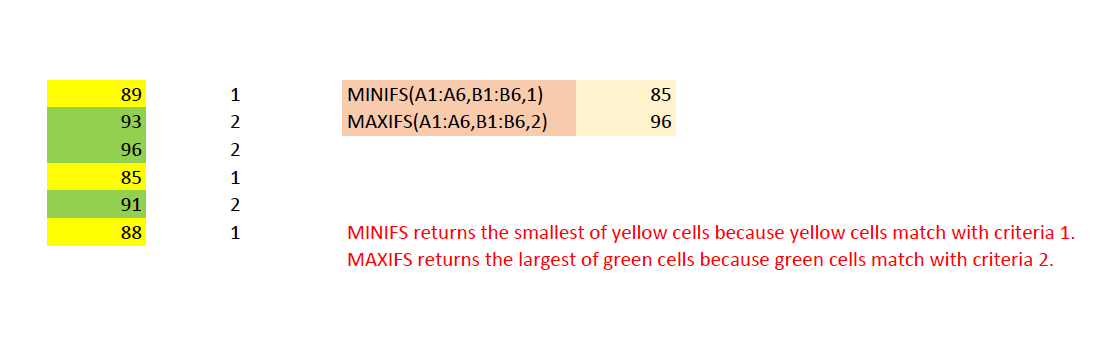# Calculation of Excel 2016 MINIFS and MAXIFS functions

Contents
[ ]

## Possible Usage Scenarios

Microsoft Excel 2016 supports MINIFS and MAXIFS functions. These functions are not supported in Excel 2013 or earlier versions. Aspose.Cells also supports the calculation of these function. The following screenshot illustrates the usage of these functions. Please read the red comments inside the screenshot to know how these functions work.## Calculation of Excel 2016 MINIFS and MAXIFS functions

The following sample code loads the sample excel file and calls the Workbook.calculateFormula() method to perform the formula calculation via Aspose.Cells and then saves the results in the output PDF.